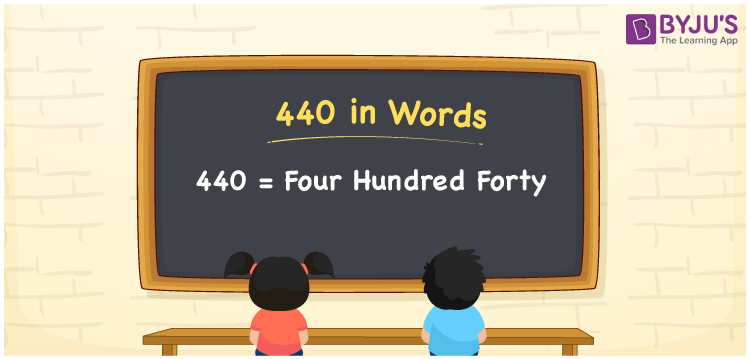# 440 in Words

The number 440 stands for “Four Hundred Forty”. The number 440 is a cardinal number, which means it can be used to count items and specify values. The number 440 can be converted to words using the place value system. If a shop has 440 flowers, you can write “A shop has four hundred forty flowers”. Let us take a closer look at the spelling of 440 in words as well as the technique in this article.

 440 in Words: Four Hundred Forty. Four Hundred Forty in Numerical Form: 440.

## 440 in English Words

The number 440 in English words is “Four Hundred Forty”.## How to Write 440 in Words?

We can utilize the place value system to convert the number 440 in words. The place value table for the number 440 is shown below.

 Hundreds Tens Ones 4 4 0

The expanded form of 440 is as follows:

= 4 × Hundred + 4 × Ten + 0 × One

= 4 × 100 + 4 × 10

= 400 + 40

= 440

= Four hundred forty

Hence, 440 in words is four hundred forty.

The number 440 is a natural number that lies between 439 and 441.

440 in words – Four hundred forty

Is 440 an odd number? – No

Is 440 an even number? – Yes

Is 440 a perfect square number? – No

Is 440 a perfect cube number? – No

Is 440 a prime number? – No

Is 440 a composite number? – Yes

## Frequently Asked Questions on 440 in Words

Q1

### How to write 440 in words?

440 in words is four hundred forty.

Q2

### Simplify 400 + 40, and express the sum in words.

Simplifying 440 + 40, we get 440. Hence, 440 in words is four hundred forty.

Q3

### Is 440 an even number?

Yes, 440 is an even number.